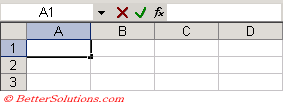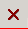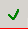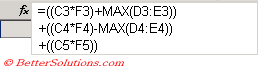### Formulas

A formula is basically a simple mathematical calculation similar that you want to be evaluated. All formulas must start with an equal sign (=) .
The simple formulas contain mathematical operators which are just symbols to denote the mathematical operations.
The most common operators are addition, subtraction, multiplication and division.
Formulas are cell entries that perform calculations and return a result.
Using formulas can be useful if you want to perform calculations on values in other cells.
This can be very useful especially if the values in other cells are likely to change.
You can either use the Formula Bar, which is located above the column headings or you could use the mouse to select the various cells.
The total number of characters in a formula cannot exceed 1,024.

#### Formulas - Complete List

This Complete List of Formulas provides details and examples.

#### Functions - Complete List

This Complete List of Functions provides details and examples for all 471 functions.

#### Using the Formula Bar

This is located below the toolbars and contains a Name box and Formula bar.
The Formula bar is the area where you can enter text, numbers, dates, formulas etc.Cancel - Cancels the immediate changes made to the active cell.Enter - Enters the number, text or formula into the active cell.

The Name box displays the name of the active cell or selected cells and can be used to apply a name to a particular cell range.
The drop-down menu next to the name box can be used to quickly navigate to particular named ranges.
As you type your formula the text will appear in both the cell and the formula bar.

#### A few facts about formulas

A formula cannot include more than 1,024 characters.
If you have formulas linking to a workbook and when this workbook is open you press (File > SaveAs) to create a backup, you will automatically change the link formulas to refer to this new file.
It is possible to enter fractions but always precede with a space to ensure that Excel does not interpret it as a date.
When entering your formulas you can press F3 when you are in the formula bar to quickly insert a named range.
To quickly copy a formula down of a cell down that have data in either of its surrounding columns just double click on the fill handle in the bottom right corner of the cell. ???

If you have really complicated formulas you may find it useful to edit the formula directly in the cell. Press F2.
What does the "(Calculate)" mean in the status bar ??

You can mess up your links by renaming the source workbook when the dependent workbook is not open.
You can easily create link formulas that refer to cells in other workbooks. If the workbook name in the reference included one or more space you must enclose it (and the worksheet name) in single quotation marks.

It is possible to enter extra spaces and carriage returns in your formulas to make them easier to read.
You can enter a carriage return by pressing (Alt + Enter).#### Formula Auto correct

Excel identifies and suggests corrections for 15 of the most common formula errors. If an incorrect formula has been entered, then a message box will appear with the option to accept or cancel the correction.
You can construct formulas using natural references as well as using named ranges.

(Ctrl + "~") - You can toggle between displaying the values and formulas by pressing
Alternatively you could press (Tools > Options)(View, Formulas)

#### Highlighting all cells that contain a formula using Conditional Formatting

This method uses the XLM language which is really old ??
Enter the formula =GET.CELL(48,INDIRECT("rc",FALSE) in the reference field of the Define Name and call the named range "FormulaInCell"
This can then be used as a condition in the Conditional Formatting

#### Fixing formula problems

Whenever you insert or move rows and columns at the edge of cell ranges referred to by formulas, the formulas are adjusted automatically.
To help you identify these problems small triangular indicators will appear in the upper left corner of a cell if Excel thinks they may be a problem. When you select the cell a smart tag will appear to display the "Formula Omits Adjacent Cells" menu.

#### Solving a set of simultaneous equations

Start with equations that are linearly independent so there is a solution
17 = 5x + 3y + 2z
13 = 2x + 4y + z
22 = 3x + 2Y + 5z
Put the coefficients of the unknowns in, e.g. "A1:C3" (i.e. 5,3,2 in A1:C1; 2,4,1 in A2:C2 and 3,2,5 in A3:C3)
Put the constants (17,13,22) in D1:D3
Highlight E1:E3 and enter "=MMULT(MINVERSE(A1:C3),D1:D3)" as an array (press Ctrl + Shift + Enter)
and the solution vector (1,2,3) will appear in E1:E3 meaning x=1, y=2, z=3
If the set of equations does not have a solution then "#VALUE" will appear
This formula returns an array of only the unique items from an expanding column
list "=IF(T(OFFSET(TheList,sArray,,1))="",N(OFFSET(TheList,sArray,,1)),T(OFFSET(TheList,sArray,,1)) )"
where TheList = OFFSET(Sheet1!\$A\$1,,,COUNTA(Sheet1!\$A:\$A),)
and sArray = SMALL(IF(MATCH(TheList,TheList,0)=ROW(TheList),ROW(TheList),""),ROW(INDIRECT(!1:"&SUM(N(MATCH(TheList,TheList,0)=ROW(TheList))))))-1"
Note that this is too slow for arrays > 1000 items

#### Maximum Size of a Formula

Formulas cannot contain more than 1,024 characters
For example the following formula contains 1,030 characters
SS

#### Extract Values from Corrupted Workbooks

If you are unable to open an Excel workbook you may be able to extract some (or all) of the data by using a linked formula.
Open a blank workbook and enter the following formula in cell A1
=[C:\temp\workbookname.xls]Sheet1!A1
Copy this formula down and across to recover as much as possible.

#### Important

It is not possible to use any of the auditing features if the worksheet is protected.
Any tracer arrows are lost when a workbook is saved or closed. The arrows will also disappear if you insert or delete any rows, move, insert or delete any cells used in the formulas or if you change the formula (any formula ?).
All formulas must start with an equal sign (=). If you type an equal sign into a cell, Excel assumes that you are about to enter a formula. If you do not enter an equal sign then Excel will assume you want to enter a text string.
Formulas can contain simple mathematical operators, values, cell references and functions and can contain up to a maximum of 1024 characters.
There is a strictly order of precedence among the operators which means that certain operations are performed before others.
Any expression in a formula that is enclosed within a parentheses is calculated first.
When copying formulas Relative cell references is the default.
Workbooks that contain a large number of formulas require a large amount of memory and hence take longer to open.
Formulas that contain links (especially to other workbooks) can often cause a real headache.

© 2019 Better Solutions Limited Next